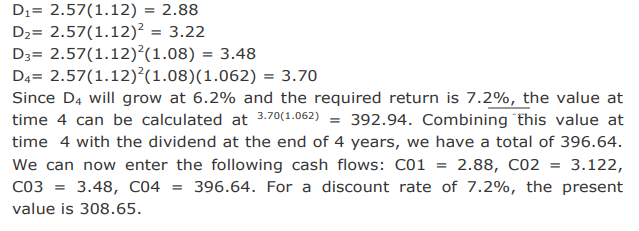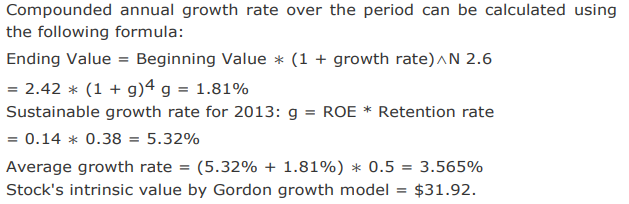# Equity Investments - Equity Investments Section 2

>>>>>>>>Equity Investments Section 2

• A

376.17.• B

388.22.• C

308.65• Option : C
• Explanation :• A

\$56.38.• B

\$66.53.• C

\$45.63.Year 2013 2012 2011 2010 2009 DPS (\$) 2.6 2.55 2.51 2.48 2.42
A company analyst uses the average of the compounded annual growth rate over the 4-year period and the sustainable growth rate for 2013 in order to estimate the growth rate of the company.She then uses the Gordon growth model to find the value of company’s stock.
Given that the required rate of return is 12%, company’s ROE in 2013 is 14% and the earnings retention rate is 38%, the stock’s intrinsic value is closest to:

• A

\$25.98.• B

\$30.82.• C

\$31.92.• Option : C
• Explanation :• Option : B
• Explanation : Required rate of return is assumed to be constant in the Gordon growth model and thus is not expected to change.

• A

Expected constant growth rate of dividends.• B

Earnings per share.• C

Share price.• Option : A
• Explanation : The firm’s fundamental leading P/E Ratio is given by: Expected dividend payout ratio Required rate - growth rate of dividends Expected dividend payout ratio may be calculated by: 1– retention ratio. Therefore, the only bit of information needed for computation is expected constant growth rate of dividends.